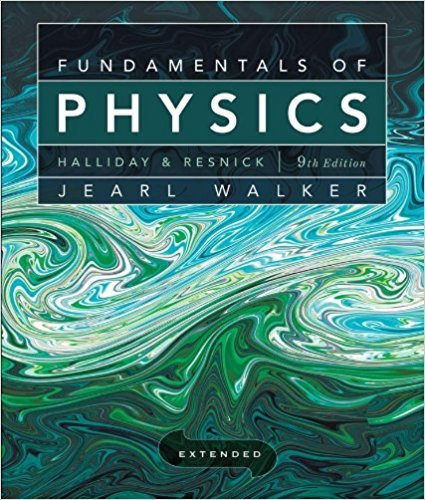×
×

# Solved: When a system is taken from state i to state fISBN: 9780470469088 189

## Solution for problem 47 Chapter 18

Fundamentals of Physics Extended | 9th Edition

• Textbook Solutions
• 2901 Step-by-step solutions solved by professors and subject experts
• Get 24/7 help from StudySoup virtual teaching assistantsFundamentals of Physics Extended | 9th Edition

4 5 1 298 Reviews
27
1
Problem 47

When a system is taken from state i to state f along path iafin Fig. lS-39, Q = 50 cal and W = 20 cal. Along path ibf, Q = 36 cal. (a) What is W along path ibf? (b) If W = -13 cal for the return path fl, what is Q for this path? (c) If Eint,i = 10 cal, what is Eint,P If Eint,b = 22 cal, what is Q for (d) path ib and (e) path bf?

Step-by-Step Solution:
Step 1 of 3

• Physicsisanexperimentalscience o Performobservationsofnaturalworld o Makeexplanationsforitspropertiesandbehaviors o Expresstheseexplanationsmathematically o Testandrefineexplanations...

Step 2 of 3

Step 3 of 3

##### ISBN: 9780470469088

The full step-by-step solution to problem: 47 from chapter: 18 was answered by , our top Physics solution expert on 12/27/17, 08:15PM. The answer to “When a system is taken from state i to state f along path iafin Fig. lS-39, Q = 50 cal and W = 20 cal. Along path ibf, Q = 36 cal. (a) What is W along path ibf? (b) If W = -13 cal for the return path fl, what is Q for this path? (c) If Eint,i = 10 cal, what is Eint,P If Eint,b = 22 cal, what is Q for (d) path ib and (e) path bf?” is broken down into a number of easy to follow steps, and 81 words. This textbook survival guide was created for the textbook: Fundamentals of Physics Extended, edition: 9. Since the solution to 47 from 18 chapter was answered, more than 224 students have viewed the full step-by-step answer. This full solution covers the following key subjects: . This expansive textbook survival guide covers 37 chapters, and 3413 solutions. Fundamentals of Physics Extended was written by and is associated to the ISBN: 9780470469088.

Unlock Textbook Solution